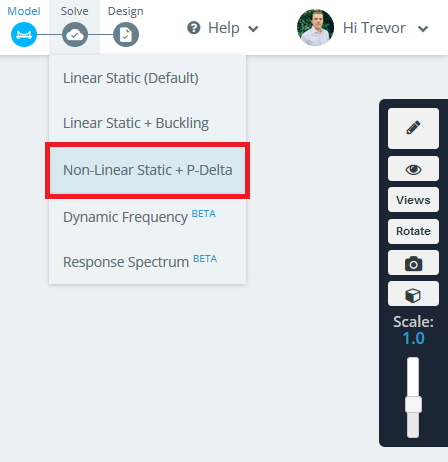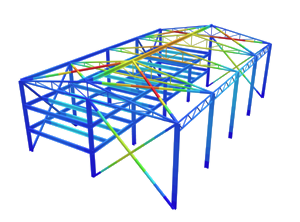SkyCiv Documentation

Your guide to SkyCiv software - tutorials, how-to guides and technical articles

1. Home
2. SkyCiv Structural 3D
3. Solving
4. Non-Linear Static & P-Delta Analysis

# Non-Linear Static & P-Delta Analysis

Non-Linear or second-order static analysis is suitable when a structure exhibits non-linear behavior. A linear analysis will be appropriate for most structures, however if the structure is geometrically non-linear then a second-order analysis is more accurate. This is especially the case if the deformation of the structure is large, as the load needs to be applied in steps. This is because after each step the structure has moved so much that the loads are not necessarily being applied in the same position as before.## Types of Non-Linear Analysis and Effects

In the Settings of the software you can change the Non-Linear solver settings. Choosing “Small” or “Finite” Displacement theory will have a significant effect on your results if the deflection of your structure was large.

As of release v2.1.1 when “Small” displacement theory is chosen, the non-linear analysis will include by P-Delta (P-Δ) and P-delta (P-δ) effects. If “Finite” displacement theory is selected then only P-Δ effects are considered. In both cases a P-Delta Analysis is being run.

## P-Delta Analysis

P-Delta Analysis is particularly important for laterally displacing, multi-storey, building structures experiencing a gravity load. These effects are found in the lateral resisting members of a structure, i.e., moment frames, shear walls, bracing, etc.

An example of a P-Delta effect is when a tall structure is subjected to a critical lateral displacement, and due to abrupt changes in axial force distribution, an overturning moment is introduced. It is the result of the ends of the member no longer being vertical due to the lateral deflection, which introduces the secondary moment (P×Δ).

This analysis works by first performing a linear static analysis. Next the P-Delta effects are taken into account for each member. The structure is re-analyzed and this iterative process continues until a convergence criteria is met on the displacement of the structure. The level of convergence can be set in the Settings of the software under the “Convergence Tolerance” setting and is reflected as a % error.

## Extra Notes and Considerations

• The SkyCiv Non-Linear solver only takes into account geometric non-linearities.
• This analysis can take a considerable amount of time to solve due to its iterative nature. It may take 10-15 times longer than the linear static analysis solution time.
• This analysis only applies to members and cables, NOT plates.